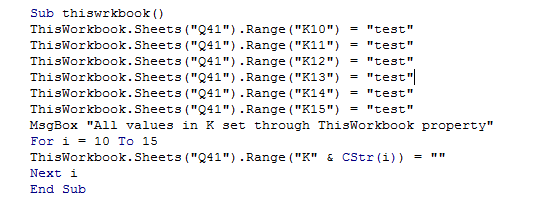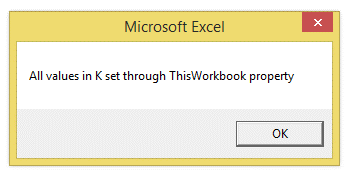## VBA thisworkbook in Excel

ThisWorkbook property returns a Workbook object that represents a workbook where the code is executing.

Thisworkbook property can be used to to refer to the workbook that contains your macro code.

Thisworkbook property is the only way to refer to an add-in workbook from inside the add-in itself.

To understand the above point, while creating an Add-in, the workbook's name may change, so by using "Thisworkbook", the same workbook is always referenced irrespective of its name.

The following example illustates this property:

1. Sub thiswrkbook()
2. ThisWorkbook.Sheets(1).Range("K10") = "test"
3. ThisWorkbook.Sheets(1).Range("K11") = "test"
4. ThisWorkbook.Sheets(1).Range("K12") = "test"
5. ThisWorkbook.Sheets(1).Range("K13") = "test"
6. ThisWorkbook.Sheets(1).Range("K14") = "test"
7. ThisWorkbook.Sheets(1).Range("K15") = "test"
8. MsgBox "All values in K set through ThisWorkbook property"
9. For i = 10 To 15
10. ThisWorkbook.Sheets(1).Range("K" & CStr(i)) = ""
11. Next i
12. End Sub

A screenshot from the editor is as shown below:The output is as shown below: